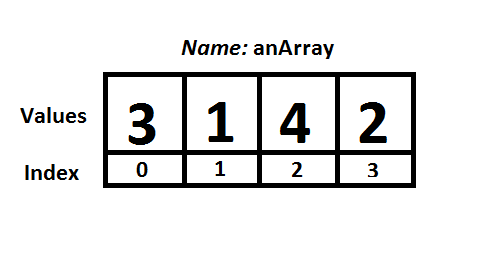# MCL Scripting: Arrays

## What is an Array?

An array is a way to store a groups of values of the same type in one continuous block of memory. The array is given an name like any other variable, but instead of only referring to one variable the array name is used to reference all of the stored values. To refer to specific locations in the array an index value, which starts at zero and end with the value one less than the size, is used to read and write to these locations.

## Creating an Array

To create an array three pieces of information must be provided: the name of the array, the type of values to store and the desired size of the array. To create an array use the following format:
arrayName VAR type(size)
anArray VAR Byte(4) ; The name of this array is “anArray”, the type is Byte (8-bit values) and the size of the array is 4
; the index values for this array are 0-3, 0 being the location of the first byte and 3 being the location of the last byte

## Reading and Writing to an Array

To read or write values to an array the name of the array and an index value are used to call out specific locations in the array. The first location in the array has an index value of zero and the last element in the array has an index value of one less than the size of the array.
anArray VAR Byte(4) ; Create the array
; writing to the array
anArray(0) = 3
anArray(1) = 1
anArray(2) = 4
anArray(3) = 2

aValue VAR Byte
aValue = anArray(0)

; printing a value from an array
puts 0,[anArray(1), 13]

## Visual Depiction of an Array

The code below creates an array and fills it with values.
; writing to the array
anArray(0) = 3
anArray(1) = 1
anArray(2) = 4
anArray(3) = 2

The illustration below depicts the layout of an array.Figure 1: A diagram of the array created by the code above.

## Iterating Over an Array

Loops in the MCL language can be used to itertate over arrays to access all of the values. Below are two examples of using different types of loop control structures to access the elements of an array. See this article for more details about the loop structures used below.

### While…Wend Iteration

; writing to the array
anArray(0) = 3
anArray(1) = 1
anArray(2) = 4
anArray(3) = 2

index VAR Byte
index = 0

while index < 4

puts 0,[anArray(index), 13]
index = index + 1

wend

### For…Next Iteration

; writing to the array
anArray(0) = 3
anArray(1) = 1
anArray(2) = 4
anArray(3) = 2

count VAR Byte

for count = 0 to 3

puts 0,[anArray(count), 13]
index = index + 1

next